Question

(a) A horizontal pipe of diameter 10 cm carries crude oil from A to C as shown in Figure 4. At a point B, midway between A and C, oil leaks at a volume flow rate of QL . The pressure drop per unit length along AB is 640 Pa/m and along BC is 600 Pa/m. Determine the leakage flow rate QL . The density of oil is 800 kg/m3 and its dynamic viscosity is 0.1 N.s/ m2 . You may assume that the flow is laminar.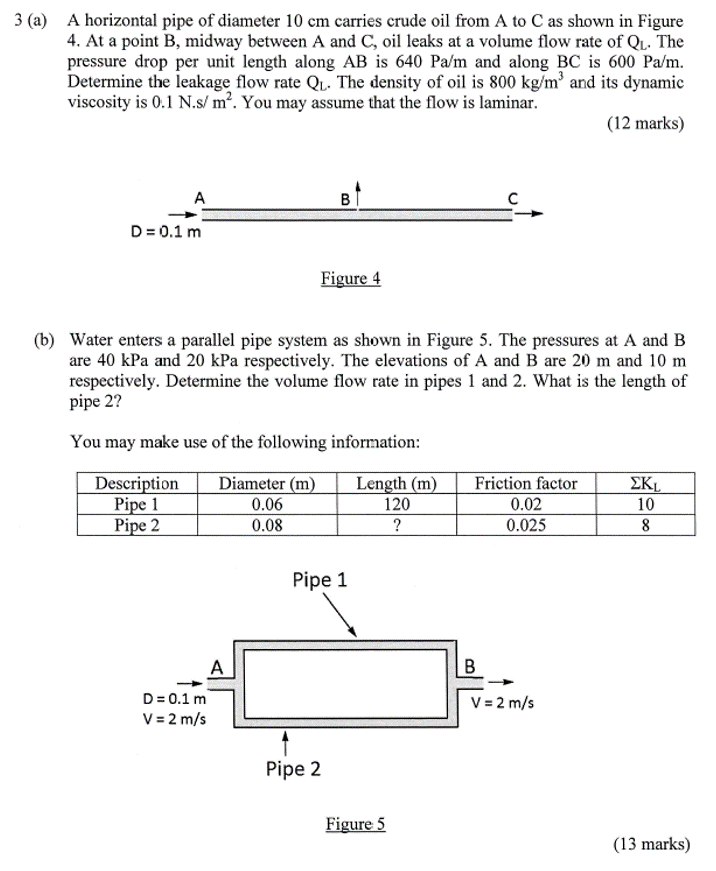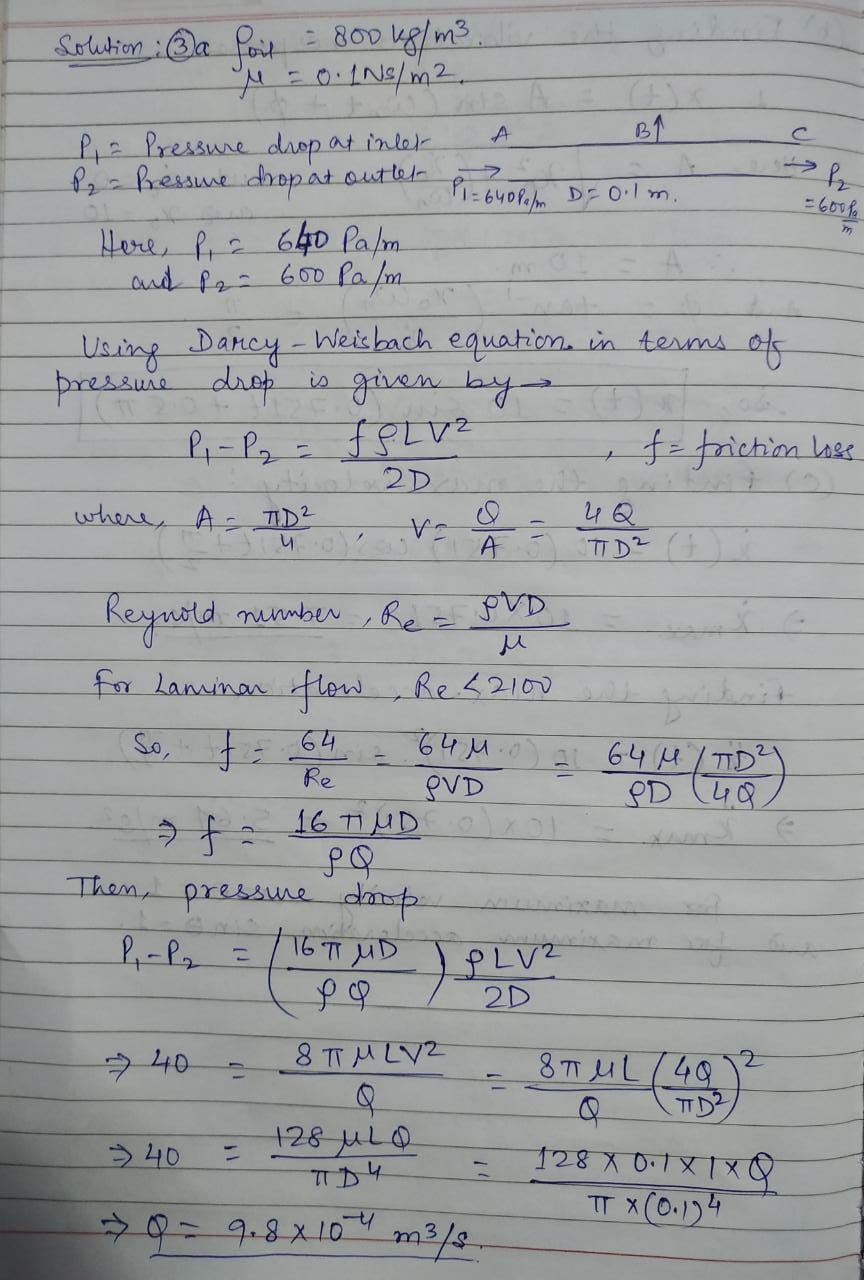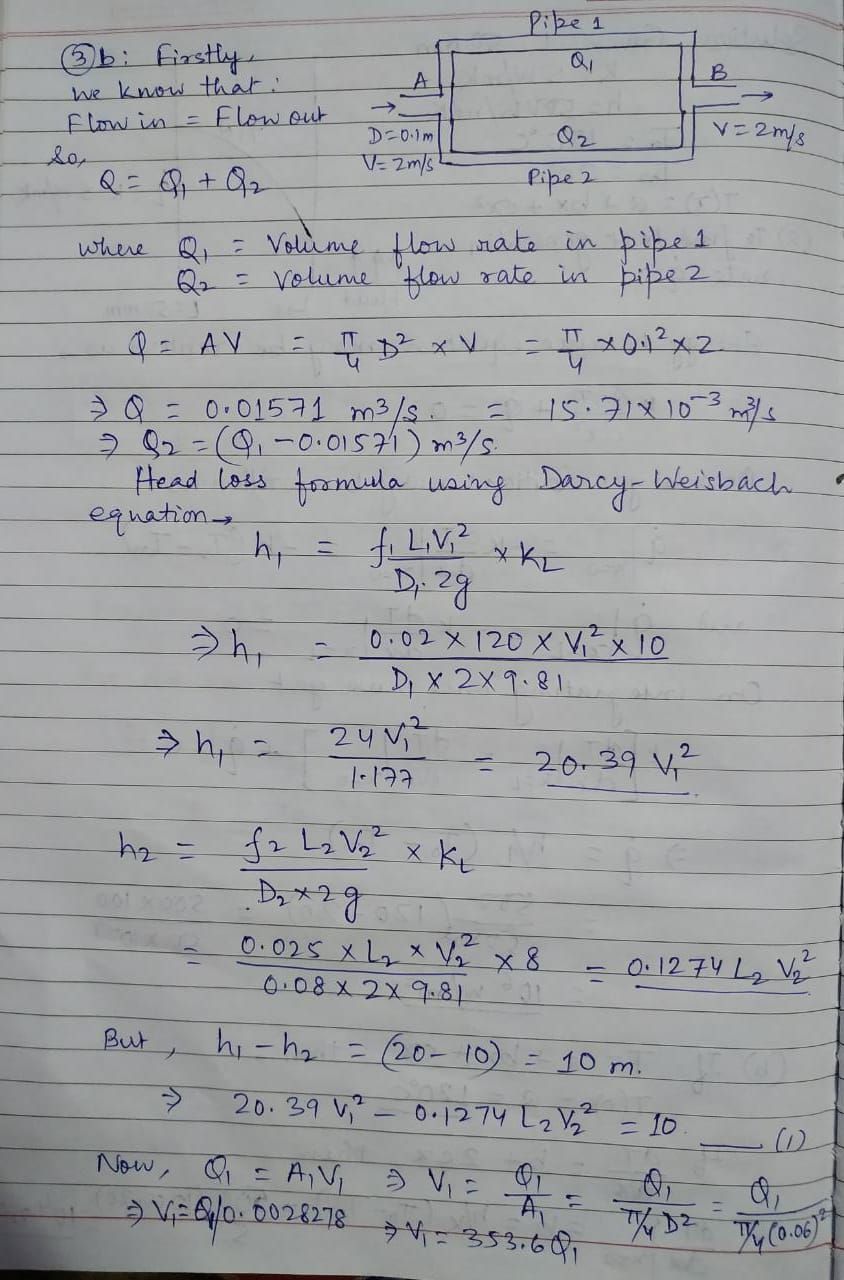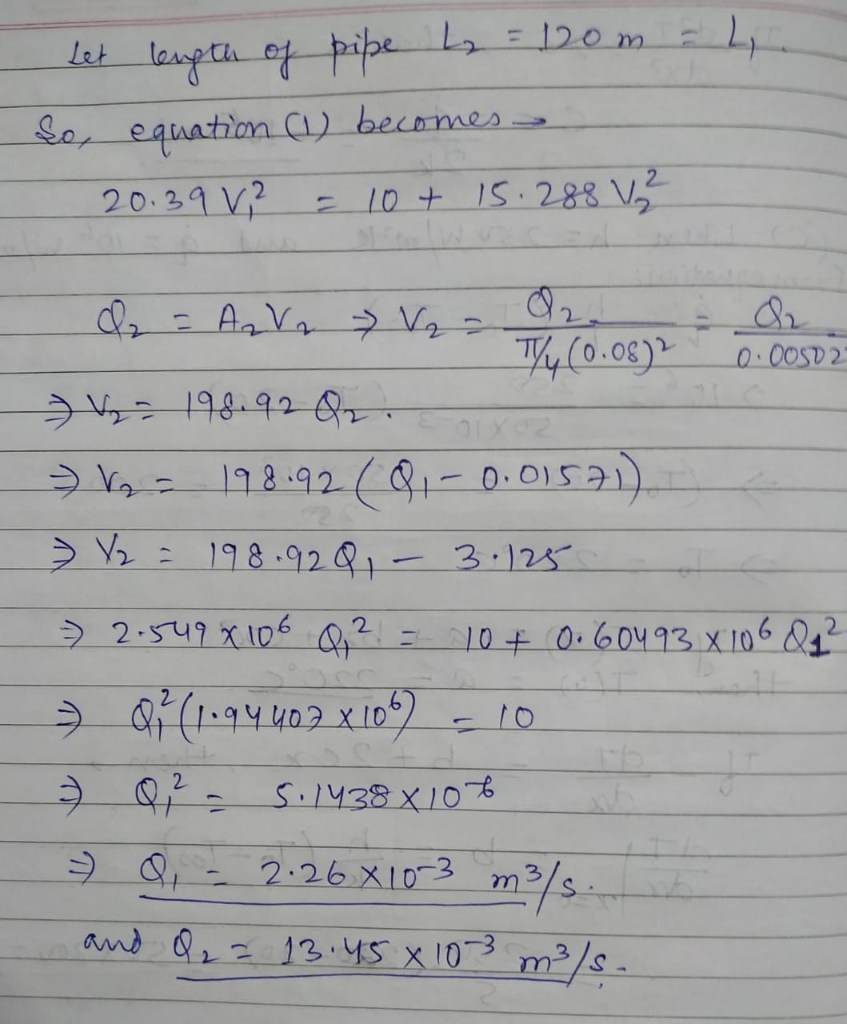Summary: Both problem is solved using Darcy- Weisbach equation.The length of pipe in question-3b will be 120m.

#### Earn Coins

Coins can be redeemed for fabulous gifts.

Similar Homework Help Questions
• ### Problem 1: A 48-cm diameter, horizontal, old commercial steel pipe, carries oil (u = 14 x...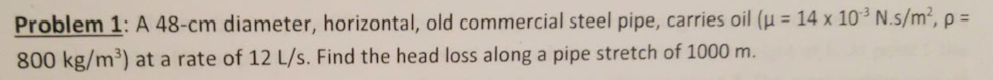Problem 1: A 48-cm diameter, horizontal, old commercial steel pipe, carries oil (u = 14 x 10 N.s/m², p = 800 kg/m?) at a rate of 12 L/s. Find the head loss along a pipe stretch of 1000 m.

• ### 84. Verify that the flow of oil is laminar (barely) an oil gusher that shoots crude oil 25.0 m into the air thro...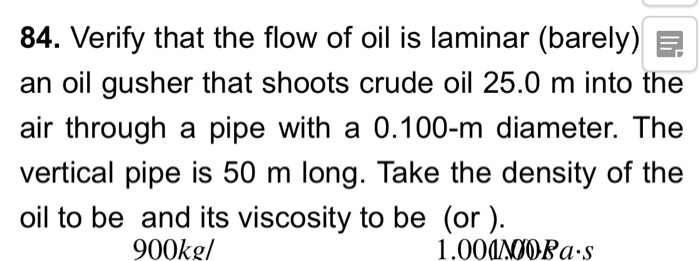84. Verify that the flow of oil is laminar (barely) an oil gusher that shoots crude oil 25.0 m into the air through a pipe with a 0.100-m diameter. The vertical pipe is 50 m long. Take the density of the oil to be and its viscosity to be (or). 900kg/ 1.00AORa.s 85. ShoW that the Reynolds number is unitless by substituting units for all the quantities in its definition and cancelling.

• ### 40 N"s/m2 and ?-1000 kg/m3 is flowing through a circular pipe umetric flow rate (Q) of...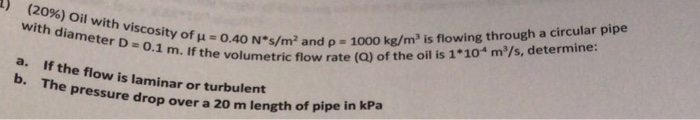40 N"s/m2 and ?-1000 kg/m3 is flowing through a circular pipe umetric flow rate (Q) of the oil is 1*10 m'/s, determine: 1) (2096) Oil with viscosity of with diameter D 0 0.1 m. If the volu a. b. If the flow is laminar or turbulent Th e pressure drop over a 20 m length of p ipe in kPa

• ### Calculate the maximum volume flow rate of fuel oil at 45 ℃ at which the flow...

Calculate the maximum volume flow rate of fuel oil at 45 ℃ at which the flow will remain laminar in a DN 100 Schedule 40 steel pipe. Assume that the SG is 0.950 and dynamic viscosity is 3.5 × 10−2 Pa. s for fuel oil.

• ### A round pipe of varying diameter carries petroleum from a wellhead to a refinery. At the...

A round pipe of varying diameter carries petroleum from a wellhead to a refinery. At the wellhead the pipe\'s diameter is 52.9 cm and the flow speed of the petroleum is 13.7 m/s. At the refinery the petroleum flows at 5.75 m/s. What is the volume flow rate of the petroleum along the pipe and what is the pipe\'s diameter at the refinery?

• ### Problem 2: Oil at 20 °C (SG 0.88) flows in the 3-cm P= 180 kPa diameter pipe shown in the figure. For the pressure...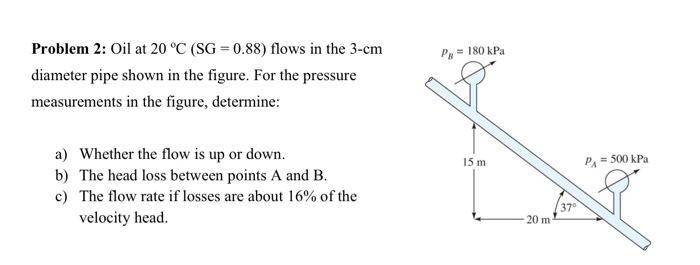Problem 2: Oil at 20 °C (SG 0.88) flows in the 3-cm P= 180 kPa diameter pipe shown in the figure. For the pressure measurements in the figure, determine: a) Whether the flow is up or down b) The head loss between points A and B P 500 kPa 15 m c) The flow rate if losses are about 16% of the 370 velocity head 20 m Problem 2: Oil at 20 °C (SG 0.88) flows in the 3-cm P=...

• ### Water flows from left reservoir to right reservoir in a 5 cm diameter pipe system as...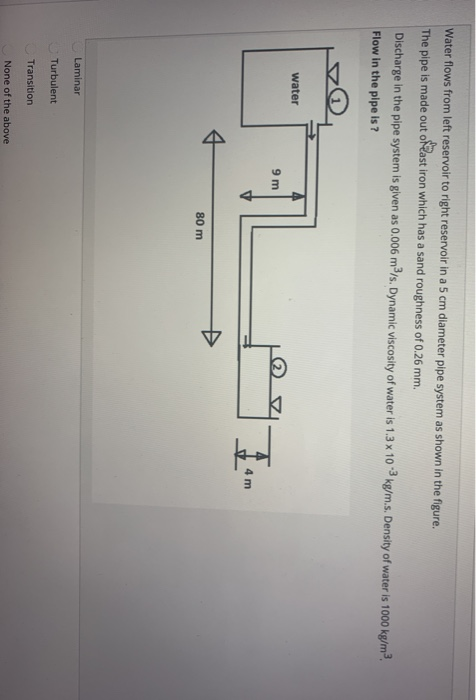Water flows from left reservoir to right reservoir in a 5 cm diameter pipe system as shown in the figure. The pipe is made out oftast iron which has a sand roughness of 0.26 mm. Discharge in the pipe system is given as 0.006 m3/s. Dynamic viscosity of water is 1.3 x 103 kg/m.s. Density of water is 1000 kg/m3 Flow in the pipe is ? water 9 m 17 4 m 80 m Laminar Turbulent Transition None of the...

• ### help Fluid enters to the section of the pipe as shown in the figure below. Density...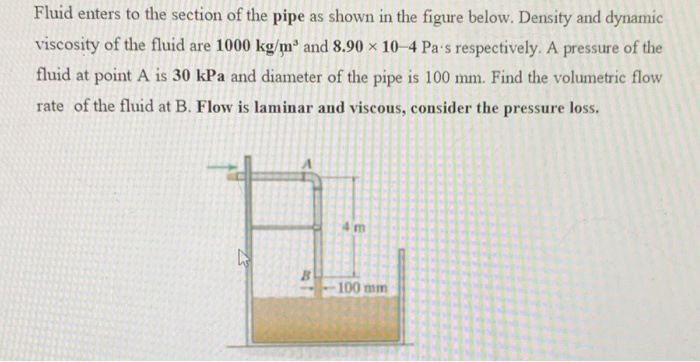help Fluid enters to the section of the pipe as shown in the figure below. Density and dynamic viscosity of the fluid are 1000 kg/mº and 8.90 x 10-4 Pa's respectively. A pressure of the fluid at point A is 30 kPa and diameter of the pipe is 100 mm. Find the volumetric flow rate of the fluid at B. Flow is laminar and viscous, consider the pressure loss. 4 m B 100 mm

• ### Question 5 a) As shown in Figure Q5 (a), crude oil at 60°C with ρ-860 kg/m3 and μ-3.91 x 10" kg s/m (about four times of viscosity of water) is pumped across from City A to City B pipeline, a...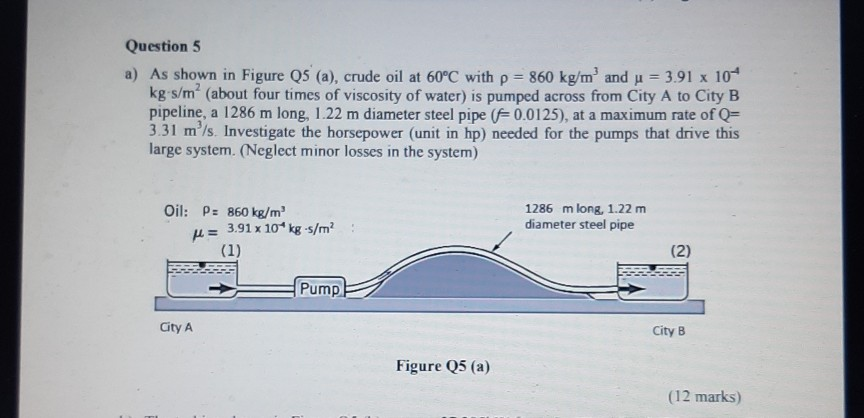Question 5 a) As shown in Figure Q5 (a), crude oil at 60°C with ρ-860 kg/m3 and μ-3.91 x 10" kg s/m (about four times of viscosity of water) is pumped across from City A to City B pipeline, a 1286 m long, 1.22 m diameter steel pipe ( 0.0125), at a maximum rate of Q- 3.31 m'ls. Investigate the horsepower (unit in hp) needed for the pumps that drive this large system. (Neglect minor losses in the system) Oil:...

• ### QUESTION 2 Calculate the head loss in an oil pipe line of length 1,976 m and...

QUESTION 2 Calculate the head loss in an oil pipe line of length 1,976 m and diameter 0.4 m for a flow rate of 1.7 l/s. Density: 854 kg/m3 ; dynamic viscosity: 0.039 kg/ (ms) Give the answer in metre fluid head to +/- 0.0001 m.